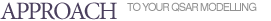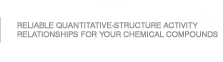GUSAR software was developed to create QSAR/QSPR models on the basis of the appropriate training sets represented as SDfile contained data about chemical structures and endpoint in quantitative terms.
QSAR MODELING ON THE BASIS OF QNA DESCRIPTORS
QNA descriptors are P and Q values calculated for each atom of molecule. The calculation of P and Q values is based on the connectivity matrix (C) and the standard values of ionization potential (IP) and electron affinity (EA) of atoms in a molecule . The estimation of a target property of chemical compound is calculated as the mean value of the function of P and Q values of the atoms of a molecule in QNA descriptors' space. We have proposed to use two-dimensional Chebyshev polynomials for approximation of the function of P and Q values. So, the independent regression variables are calculated as average values of particular two-dimensional Chebyshev polynomials of P and Q values for molecule atoms.

QNA descriptors and their polynomial transformations do not provide information on the shape and volume of a molecule although this information may be important for determining the structure-activity relationships. Therefore, these parameters were added to the variables obtained from Chebyshev polynomials. Topological length of the molecule is the maximal distance, calculated by the number of bonds between any two atoms (including hydrogen). The volume of a molecule is the sum of each atom's volume.

The number of initial variables for QSAR modelling depends on the number of compounds in the training set and corresponds to the number of Chebyshev polynomials plus the number of the first, second and third power of the values of topological length and volume of a molecule.

GUSAR algorithm uses three randomly selected parameters to generate different QSAR models based on QNA descriptors: (a) calculation of QNA descriptors for all atoms or for the atoms in a molecule with two or more immediate neighbours; (b) changing of the coefficient before the connectivity matrix (c) changing of parameters of Chebyshev polynomials.